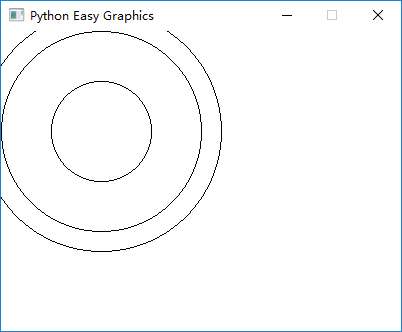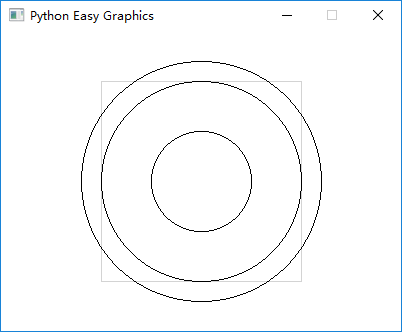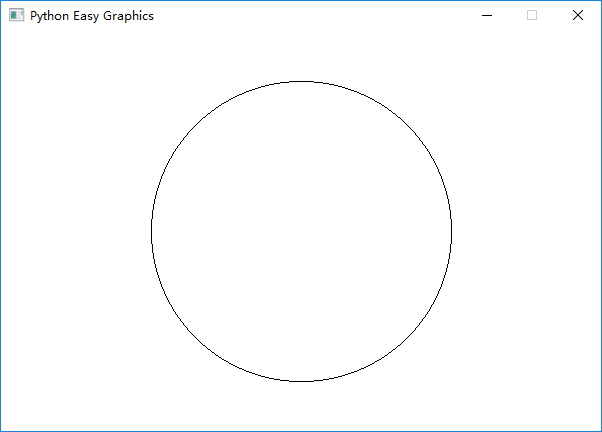# 视口（View Port）与逻辑窗口（Logical Window）¶

## 视口（View Port）¶

```from easygraphics import *

def main():
init_graph(400, 300)
circle(100, 100, 50)
circle(100, 100, 100)
circle(100, 100, 120)
pause()
close_graph()

easy_run(main)
``````from easygraphics import *

def main():
init_graph(400, 300)
set_color("lightgray")
draw_rect(100, 50, 300, 250)
set_color("black")

set_view_port(100, 50, 300, 250)
circle(100, 100, 50)
circle(100, 100, 100)
circle(100, 100, 120)
pause()
close_graph()

easy_run(main)
``````from easygraphics import *

def main():
init_graph(400, 300)
set_color("lightgray")
draw_rect(100, 50, 300, 250)
set_color("black")

set_view_port(100, 50, 300, 250, clip=False)
circle(100, 100, 50)
circle(100, 100, 100)
circle(100, 100, 120)
pause()
close_graph()

easy_run(main)
```## 逻辑窗口¶

**物理坐标系**是实际在绘图设备（绘图窗口或者图像画布）上的坐标系。原点(0,0)永远位于设备的左上角，X轴正向朝右，Y轴正向向下。

**逻辑坐标系**是我们在绘图指明图形位置时所用的坐标。例如，我们执行circle(50,50,100)时，圆心(50,50)就是逻辑坐标。

Easygraphics在绘图时，会将逻辑坐标值转换为物理坐标值。在缺省状态下，逻辑坐标系和物理坐标系是重合的。而视口和逻辑窗口的设置会影响如何进行坐标转换。

Qt的文档 详细说明了坐标系转换的过程，请参阅。

• 说明1：我们设置的视口是600像素宽，400像素高；逻辑窗口的宽是6，高是4。因此，在x轴方向上我们得到了600/6=100倍放大；在y轴方向我们也得到了400/4=100倍放大。
• 说明2：逻辑窗口的宽是6，高是4，并且我们把逻辑窗口的左上角放到了(-3,-2)，因此(0,0)就到了窗口的正中。
```from easygraphics import *

def main():
init_graph(600, 400)
set_window(-3, -2, 6, 4)

circle(0, 0, 1.5)
pause()
close_graph()

easy_run(main)
```